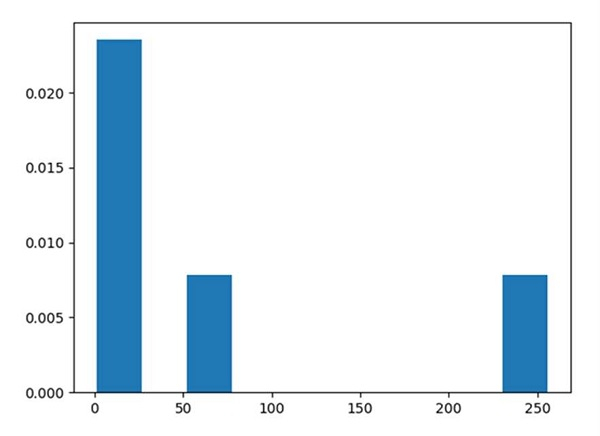# How can I plot a histogram such that the heights of the bars sum to 1 in matplotlib?

In plt.hist() method, stacked=True could help to get the heights of the bars sum to 1.

## Steps

• Create a list of numbers.

• Using plt.hist(), we can draw the histogram.

• stacked : bool, default: False

If "True", multiple data are stacked on top of each other If False multiple data are arranged side by side if histtype is 'bar' or on top of each other if histtype is 'step'.

• density : bool, default: False

If "True", draw and return a probability density: each bin will display the bin's raw count divided by the total number of counts *and the bin width*.

• To show the figure, use the plt.show() method.

## Example

from matplotlib import pyplot as plt

x = [1, 4, 16, 64, 256]

# stacked and density are true then the sum of the histograms is normalized to 1.
plt.hist(x, 10, stacked=True, density=True)
plt.show()

## Output